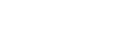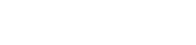## Chemistry Paper 1 Questions and Answers - Alliance Mock Examinations 2022

QUESTIONS

1.
1. State and explain the observation made when sodium carbonate powder is added to Aluminum Chloride solution. (2 marks)
2. Identify the acid in the forward reaction given the equation below explain.
NH4+(aq) + OH-(aq)  NH3(g) + H2O(I)
2. Describe how you would prepare a sample of Barium Sulphate using the following reagents; Dilute Sulphuric (VI) acid, dilute Hydrochloric acid and Barium Carbonate. (3 marks)
3. Copper (II) Sulphate reacts with barium Chloride according to the equation below. CUSO4(aq) + BaCl2(aq)→CuCl2(aq) + BaSO4(s) ΔH=-17.7
Calculate the temperature change when 900cm3 of IM copper(II) Sulphate is added to 600cm3 of 1M barium Chloride. (C-4.2J-'K', density of solution 1g/cm3)(3 marks)
4. An element T forms a divalent cation with electronic arrangement of 2,8,8.
1.
1. To what period does it belong? (1 mark)
2. Name the chemical family to which it belongs.
2. Write the formula of its Nitride.
5. The set-up below was used to investigate a property of ammonia gas.1. Explain the observation made at B.
2. Write the chemical equation for the reaction at A.
6. Barium Sulphite was added to Equal volumes of equimolar monobasic acids L and M in separate test tubes.
The table below shows the volumes of gas produced after sometime.
 Acid Volume of gas (cm3) L 4 M 10
(1 mark)
1. Define basicity. (1 mark)
2. Propose an explanation for the observations made. (1 mks)
3. Give one possible identity of M.
7. A organic compound N with the molecular formula C4H10O reacted with a piece of sodium metal to produce hydrogen gas and a colourless solution.
1. To which homologous series does N belong? (1 mark)
2. State the observations made. (1 mark)
3. Write a chemical equation for the reaction that occurred. (1 mark)
8. 48cm3 of methane was ignited with 212cm3 of oxygen and the mixture allowed to attain room temperature. Determine the total volume of the resultant gaseous mixture. (3 marks)
1. Complete the nuclear equation for the reaction below. (1 mark)2.
1. State one use of radioisotopes in agriculture. (1 mark)
2. Give one danger associated with exposure of humans to radioisopes (1 mark)
9. Study the flow chart below and answer the questions that follow.1. Identify the type of reaction in step 1
2. Identify W & X
3. State the condition necessary in step 2 and write the equation for the reaction that occurs
10. Given the following thermochemical equations, use an energy level diagram to determine the enthalpy of formation of hydrogen peroxide. (3 marks)
H2(g) + ½O(g) → H2O(I)  ΔH=-286kJ/mol
H2O2(I) → H2O(I) + ½O(g) ΔH = -98 kJ/mol.
11. The flow chart below shows the extraction of Zinc metal from its chief ore. Study the flow chart and answer the questions that follow.1. Name and write the formula of the chief ore.
2. Identify A and B.
3. Write the equation for the reaction in the roasting furnace.
4. State the functions of the lead spray.
12. When 10g of a mixture of potassium chloride and anhydrous sodium sulphate is dissolved in water and excess barium chloride solution added. 6.9g of barium sulphate is precipitated. Calculate the composition of the mixture. (K 39, CI= 35.5, Na =23, S = 32, O=16, Ba=137)
13. In an experiment, a student placed equal volumes of mixtures of ethanoic acid in water (tube A) and ethanoic acid in hexane (tube B). in each test tube, 1g of solid sodium hydrogen carbonate (2 marks) was added. State and explain the observations made.
14. The following reaction is in equilibrium in a closed system.
C(s) + H2O(g)  CO(g) + H2(g)
1. What is a chemical equilibrium?
2. State L Chatelier's Principle.
3. Explain how an increase in pressure would affect the amount of hydrogen gas in the system.
15. Give the IUPAC names of the following.
1.    C2H5
I
CH3CHCCH3
2. CHCCH(CH3)CH(Br)CH3
3. CH3CHCH2OH
I
Br
16. The scheme below represents the manufacture of a cleansing agent N.1. Name reagent M.
2. What type of cleansing agent is N.,
3. Draw the structure of N.
4. State one advantage of using N as a cleansing agent.
17. The table below shows properties of some chlorides. Study it and answer the questions that follow.
 Chloride Mpt(°C) Bpt (°C) Electrical conductivity in aqueous solution pH of solution AIC13 - 183 Good NaCl 860 1420 Good PCl5 32 75 Good HCI -146 -29 Good
18.
1. Explain the high melting and boiling points of sodium chloride.
2. Draw the dot (.) and cross (x) diagram to show the bonding in HCI.
3. Explain the electrical conductivity of the aqueous solutions.
19. 100cm3 of 2M sulphuric (vi) acid was electrolyzed using the set up represented by the diagram below.1. Label the anode and the cathode.
2. Write the equation for the reaction at the anode.
3. Comment on the concentration of the electrolyte as electrolysis continues. (1 mark)
20. The diagram below is a sketch of the energy level diagram of the non-catalyzed reaction of zinc powder with dilute hydrochloric acid.1. State whether the reaction above is exothermic or endothermic. (1 mark)
2. On the same axis, sketch the graph for the reaction when copper (II) Sulphate crystals are added. Explain. (2 marks)
21.
1. State Graham's Law of diffusion. (1 mark)
2. 100cm3 of Sulphur (IV) Oxide takes 20 seconds to diffused through a porous plate. What volute of oxygen gas would diffuse through the same plate in 30 seconds under similar conditions. (S=32,0=16) (2 marks)
22. Below is a list of standard reduction potentials for two half-cells in acidic conditions. Use them to answer the questions that follow.
MnO2(s) + 4H+(aq) + 2e- ⇌ Mn2+(aq) + 2H2O(I) + 1.23
Cl2(g) +2e + 2Cl-(aq)   + 1.36
Predict whether manganese (IV) Oxide would oxidize hydrochloric acid to chlorine gas. (2 marks)
23. Sulphur (IV) Oxide gas was bubbled into water containing litmus solution.
1. State the observation made. (1 mark)
2. Explain the observation in (1) above with the Grid of equations. (2 marks)
24. lon exchange is a method used to remove water hardness. Study the diagram below and answer the questions that follow.Card A: ion exchange                                Card B: lan exchange column
Column before hard                            after hard water is passed through
Water is passed through
1. What causes water hardness
2. Fill in carb B.
3. Other than lon exchange, state two methods used to remove both temporary and permanent water hardness.
25.
1. Describe how carbon (IV) Oxide can be distinguished from carbon (11) Oxide using calcium hydroxide solution.
2. What is the role of carbon (IV) Oxide in fire extinguishing.
26. In an experiment, dry hydrogen chloride gas was passed through heated zinc turnings as shown in the diagram below. He gas produced was then passed through heated lead (II) Oxide.1. State the function of the water in the flask.
2. Write the equations for the reactions that took place in tube D and B.## MARKING SCHEME

1.
1. Effervesence of a colourless gas, sodium carbonate dissolves; colourless solution formed
AlCl3 is hydrolysed in water forming an acidic solution
2. NH4+(aq)2, proton donor
2. Add excess BaCO3(s) to dil. HCl while sitting. Filter to obtain BaCl2(aq) to H2SO4(aq). Filter to obtain BaSO4(s) residue
Rinse with distilled water and dry between filter papers
3. CuSO4 is in excess
Moles of BaCl2 = 600  x 1 = 0.6moles
1000
Heat = 0.6 x 17.7 = 10.62kJ
1000 x 10.62 = 1500 x 4.2 x T
T = 1.7K
Tempeature rose 1.7ºC
4.
1.
1. 4
2. Alkaline earth metals
2. T3N2
5.
1. White powder changes to blue crystals
Water formed changes anhydrous copper(II) sulphate to hydrated copper(II) sulphate
2. 2NH3(g) + 3CuO(s) → 3Cu(s) + N2(g) + 3H2O
6.
1. The number of replacable hydrogen atoms per molecule of an acid
2. L- A weak acid, higher concentration of H+ions faster rate of reaction. M is a strong acid
3. HCl , H2SO4, HNO3
7.
1. Alkanols
2. Na(s) sinks to the bottom
Colourless gas bubbles produced
3. 2C4HaOH + 2Na → 2C4HaONa + H2
8. CH4(g) + 2O2(g) → CO2(g) + 2H2O(I)
48cm3  96cm3  48cm3
Excess O2 = 212 - 96 = 116cm3
Total = 48 + 116 = 164cm3
1.2.
1. Radioactive to determine rate of absorption of phosphate fertilizer
Radioactive C-14 used in monitoring photosynthesis
2. Gene mutation, cancer, death
9.
1. Decarboxylation
2. W - Sodium propate
X - Ethane
3. U.V light
C2H6 + 6Cl2 → C2Cl6 + 6HCl
10.11. ΔHf(H2OS) = (-286) +
(+98)
= -188kJ/mol
1. Zinc blende
ZnS
2. K - SO2(g)
B - Coke and limestone
3. 2ZnS(s) + 3O2(g) → 2ZnO(s) + 2SO(g)
4.
1. Cools zinc vapour, to condense to Zn(I)
2. Prevents the Zn from being re-oxidized
12. BaCl2(aq) + Na2SO4(aq) → BaSO4(s) + NaCl(aq)
0.0296moles
Mass of Na2SO4 = 0.0296 x 142
= 4.2032g
4.2032g NaSO4
5.798g KCl(s)
13. Tube A - colourless gas bubbles, white solid dissolves to form colourless solution. Acid ionises in water
Tube B - No effervescence, white solid does not dissolve. Acid does not ionise in hexane, No H+(Aq)
14.
1. A state of a closed chemical system in which the rate at which reactants are converted to products equals the rate at which products are converted to reactants
2. When a change in conditions is applied to a system in equilibrium, the system moves so as to oppose the change
3. reduces; reverse reaction produces fewer moles of gas and is favoured
15.
1. 3 -methyl pent-2-ene
2. 4-bromo-3-methyl pent-1-yne
3. 2-bromo propan-1-0
16.
1. Concentrated sulphuric (vi) acid
2. soapless detergent
3.4. lathers readily with both soft and hard water
17.
18.
1. giant ionic structure with strong ionic bonds
2.3. mobile ions
19.
1.2. 4OH-(aq) → 2H2O(I) + O2(g) + 4e-
3. Increases, 2H+ and OH from water are discharged
20.
1. exothermic
2. catalyst provides alternative route that requires lower activation energy
21.
1. Under the same conditions of temperature and pressure the rate of diffusion of a gas is inversely proportional to the r of its density
2. RSO2 = √ MO
RO2       MSO2
100/20 = √32/64
RO2
RO= 25 x 64
32
= 7.071cm3/s x 30
= 212.13cm3
22. MnO2(s) + 4H+ + 2e → Mb2+(aq) + 2H2O(I)  +1.23V
2Cl-(aq) → Cl2(g) + 2e-                                  - 1.36V
MnO2(g) + 4H+ 2Cl(aq) → Mn2+(g) + Cl2(g) + 2H2O - 0.13V
23.
24.
1. presence of Mg2+ or Ca2+ ions
2.3.
1. Distillation
25.
1. Bubble the gases into CaOH2(aq) in separate boiling tubes
CO2 - white precipitate
CO - No white precipitate
2. Dense than air, covers the fire cutting off oxygen supply
26.
1. To absorb excess HCl(g)
2. Tube D → 2HCl(g) + Zn(s) → H2(g) + ZnCl2(s)
B - PbO(s) + H2(g) → Pb(s) + H2O(I)

• ✔ To read offline at any time.
• ✔ To Print at your convenience
• ✔ Share Easily with Friends / Students